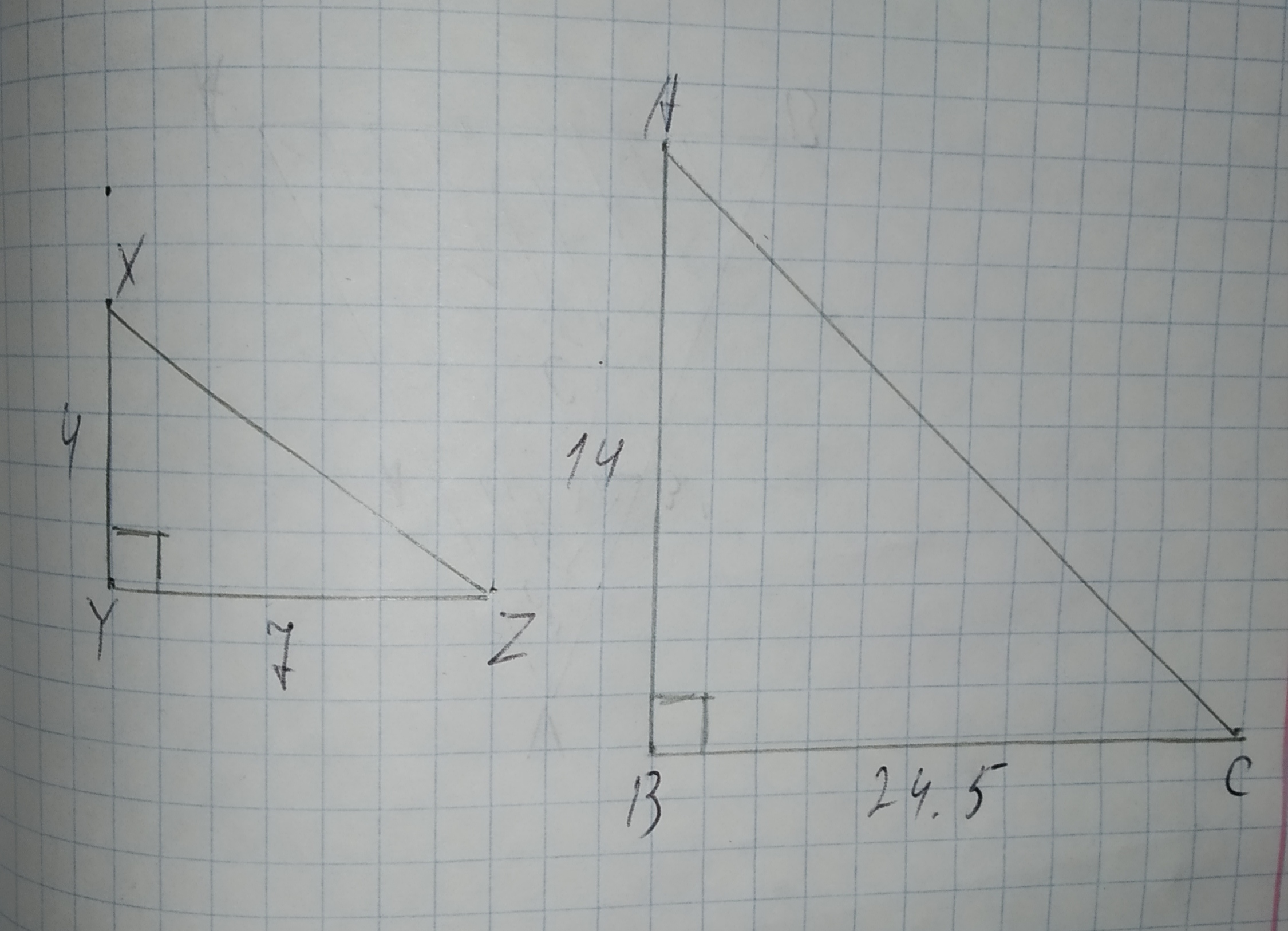# To check: whether the additional information in the given option would be enough to prove the given similarity. Given: The given similarity is /_ADC~/_BEC 12210202961.jpg The given options are: A./_DAC and /_ECB are congruent. B.bar(AC) and bar(BC) are congruent. C.bar(AD) and bar(EB) are parallel. D./_CEB is a right triangle.Question
SimilarityTo check: whether the additional information in the given option would be enough to prove the given similarity.
Given:
The given similarity is $$\displaystyle\triangle{A}{D}{C}\sim\triangle{B}{E}{C}$$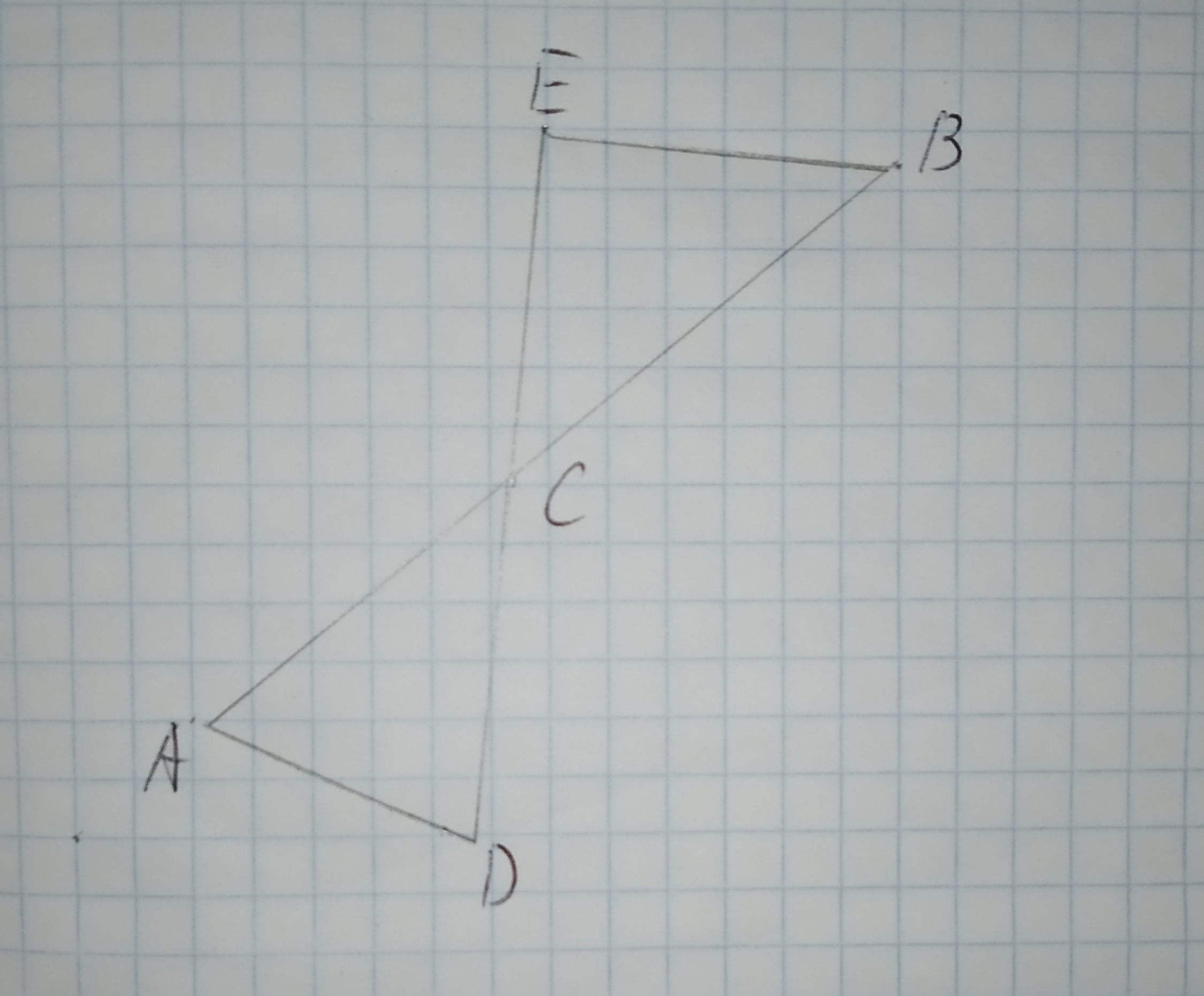The given options are:
A.$$\displaystyle\angle{D}{A}{C}{\quad\text{and}\quad}\angle{E}{C}{B}$$ are congruent.
B.$$\displaystyle\overline{{{A}{C}}}{\quad\text{and}\quad}\overline{{{B}{C}}}$$ are congruent.
C.$$\displaystyle\overline{{{A}{D}}}{\quad\text{and}\quad}\overline{{{E}{B}}}$$ are parallel.
D.$$\displaystyle\angle{C}{E}{B}$$ is a right triangle.2021-01-29
Calculation:
The given similar triangle is $$\displaystyle\triangle{A}{D}{C}\sim\triangle{B}{E}{C}$$. And this is only possible $$\displaystyle\overline{{{A}{D}}}{\quad\text{and}\quad}\overline{{{E}{B}}}$$ are parallel.
Therefore, $$\displaystyle\angle{C}{A}{D}{\quad\text{and}\quad}\angle{C}{D}{A}$$ is equal to the $$\displaystyle\angle{C}{B}{E}{\quad\text{and}\quad}\angle{C}{E}{B}$$, so that the triangle is similar by AA-similarity
Hence, the correct option is (C).

### Relevant QuestionsWhat other information do you need in order to prove the triangles congruent using the SAS Congruence Postulate?A)$$\displaystyle\angle{B}{A}{C}\stackrel{\sim}{=}\angle{D}{A}{C}$$
B)$$\displaystyle\overline{{{A}{C}}}\stackrel{\sim}{=}\overline{{{B}{D}}}$$
C)$$\displaystyle\angle{B}{C}{A}\stackrel{\sim}{=}\angle{D}{C}{A}$$
D)$$\displaystyle\overline{{{A}{C}}}\stackrel{\sim}{=}\overline{{{B}{D}}}$$Whether the triangle are similar. if so write the similarity statement and if not what would be the sufficient to prove the triangle similar.
Given: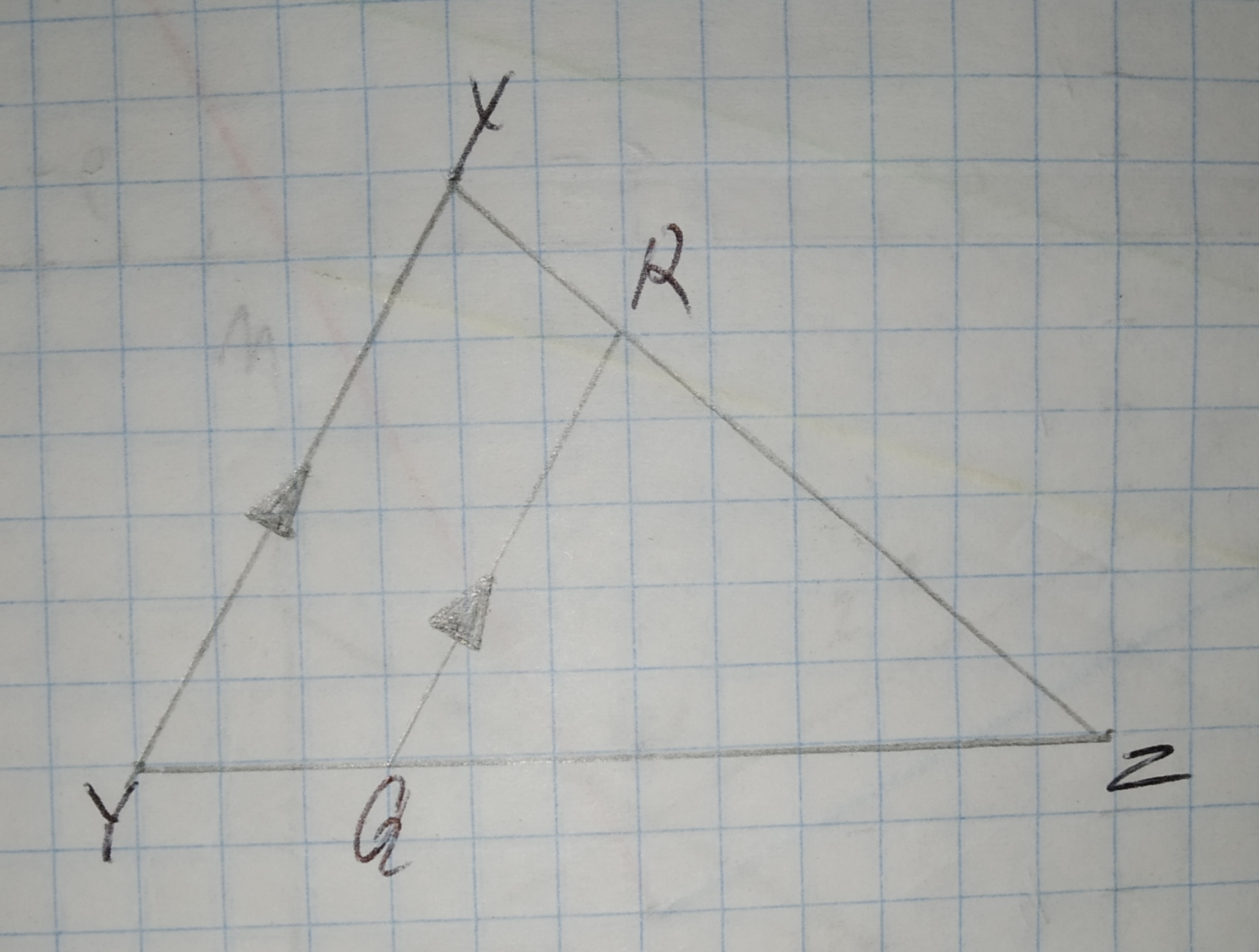Proving Similarity in the figure DEFG is a square. Prove the following:
$$\displaystyle{D}{E}=\sqrt{{{A}{D}\cdot{E}{B}}}$$
Given:
The given figure is,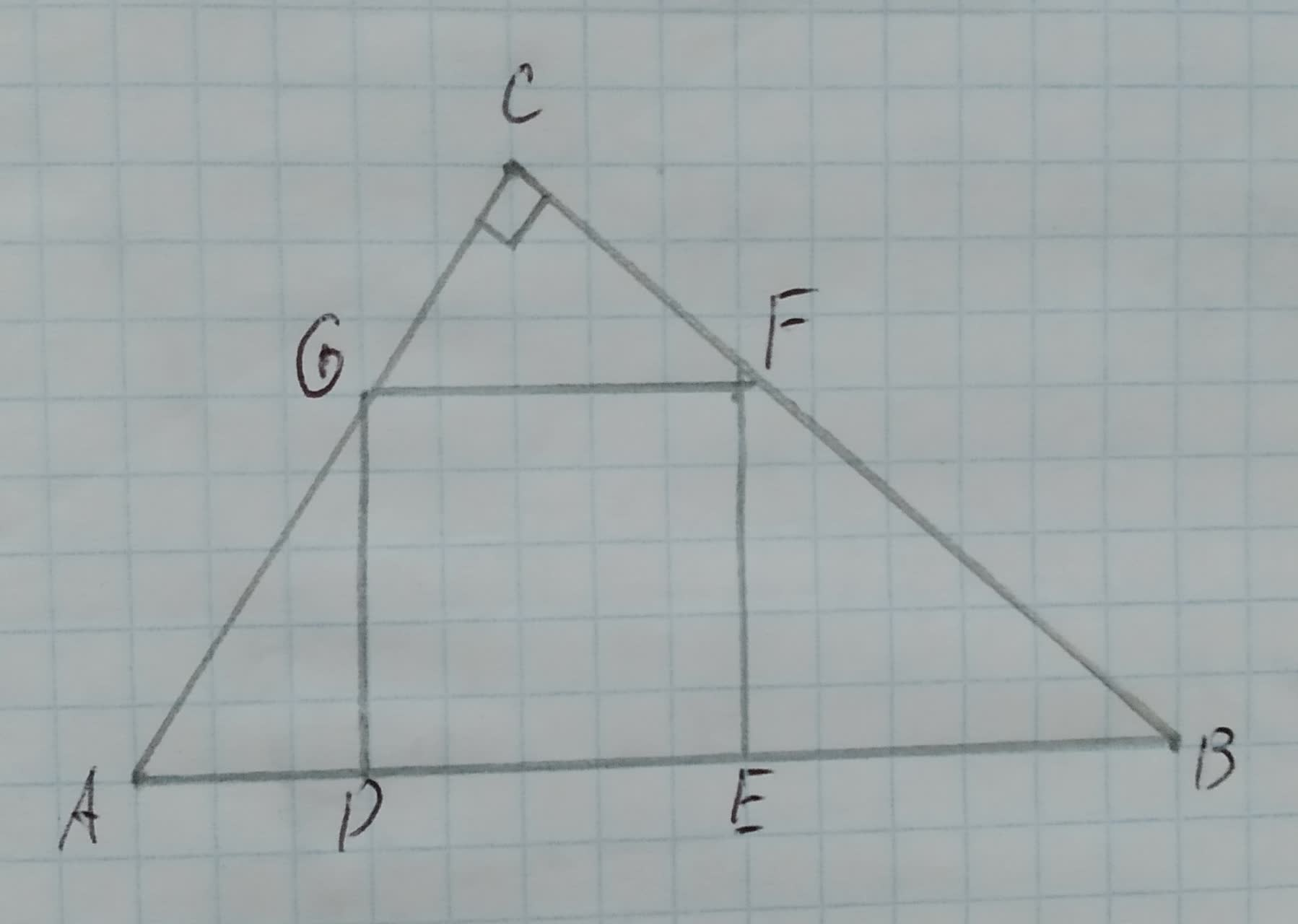Proving Similarity in the figure DEFG is a square. Prove the following:
Given:
The given figure is,Draw a graph for the original figure and its dilated image. Check whether the dilation is a similarity transformation or not.
Given:
The given vertices are
original $$\displaystyle\rightarrow{A}{\left({2},{3}\right)},{B}{\left({0},{1}\right)},{C}{\left({3},{0}\right)}$$
image $$\displaystyle\rightarrow{D}{\left({4},{6}\right)},{F}{\left({0},{2}\right)},{G}{\left({6},{0}\right)}$$Using Similarity Express x in terms of a, b, and c.
Given:
The given pair of triangles is similar.
The given pair of triangle is,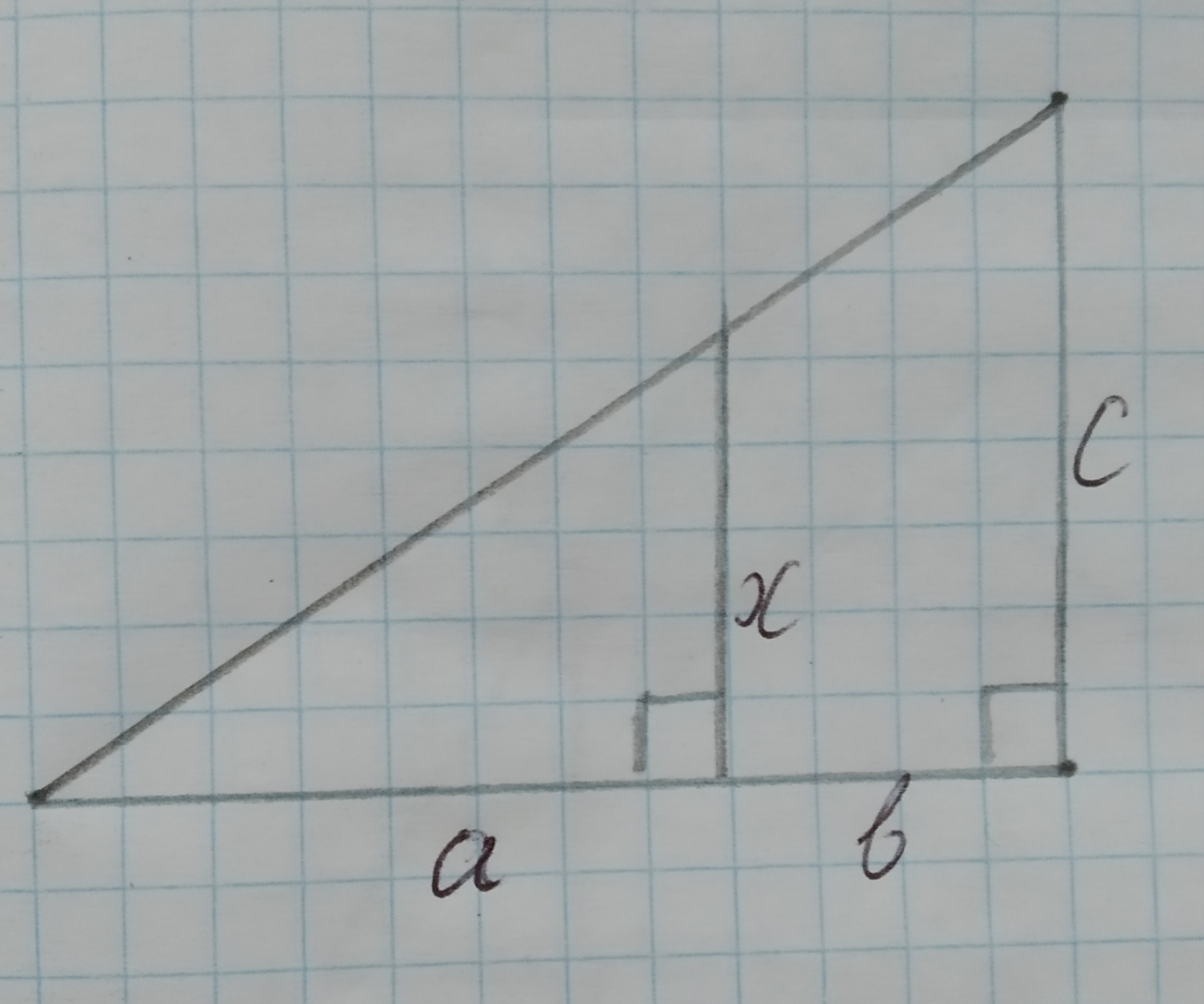To check: whether the triangles are similar. If so, write a similarity statement.
Given:
The given triangles are: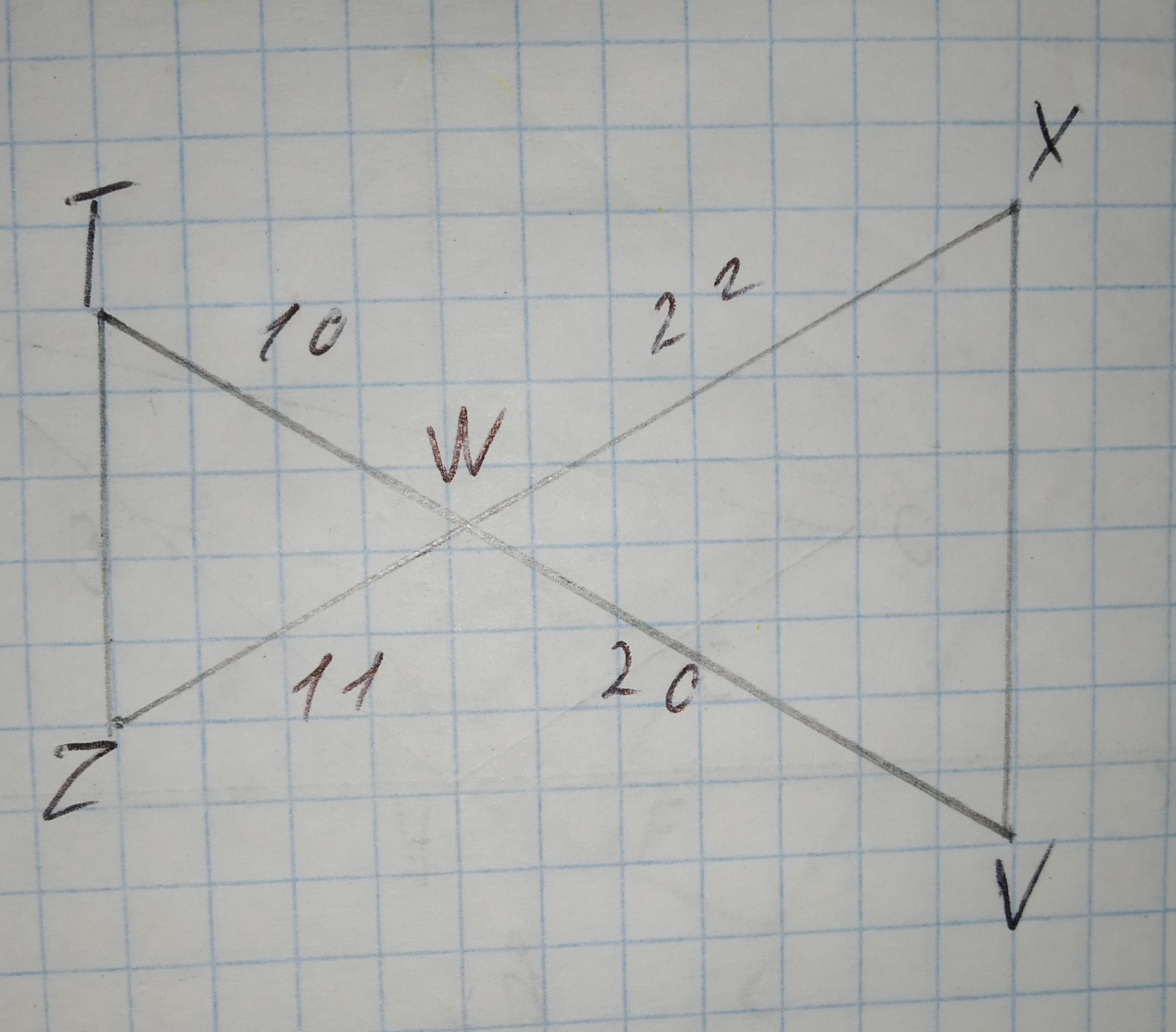Draw a graph for the original figure and its dilated image. Check whether the dilation is a similarity transformation or not.
Given:
The given vertices are
J(-6,8),K(6,6),L(-2,4),D(-12,16),G(12,12),H(-4,8)